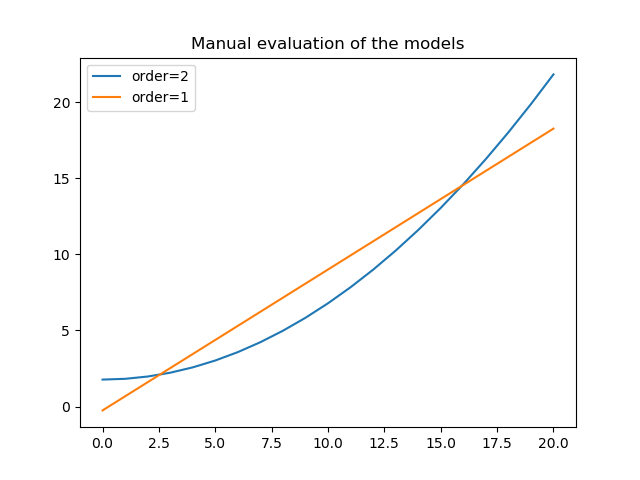# Simple Interpolation

## Overview

Although Sherpa allows you to fit complex models to complex data sets with complex statistics and complex optimisers, it can also be used for simple situations, such as interpolating a function. In this example a one-dimensional set of data is given - i.e. $$(x_i, y_i)$$ - and a polynomial of order 2 is fit to the data. The model is then used to interpolate (and extrapolate) values. The walk through ends with changing the fit to a linear model (i.e. polynomial of order 1) and a comparison of the two model fits.

## Setting up

The following sections will load in classes from Sherpa as needed, but it is assumed that the following modules have been loaded:

>>> import numpy as np
>>> import matplotlib.pyplot as plt


The data is the following:

x

y

1

1

1.5

1.5

2

1.75

4

3.25

8

6

17

16

which can be “loaded” into Sherpa using the Data1D class:

>>> from sherpa.data import Data1D
>>> x = [1, 1.5, 2, 4, 8, 17]
>>> y = [1, 1.5, 1.75, 3.25, 6, 16]
>>> d = Data1D('interpolation', x, y)
>>> print(d)
name      = interpolation
x         = Float64
y         = Float64
staterror = None
syserror  = None
None


This can be displayed using the DataPlot class:

>>> from sherpa.plot import DataPlot
>>> dplot = DataPlot()
>>> dplot.prepare(d)
>>> dplot.plot()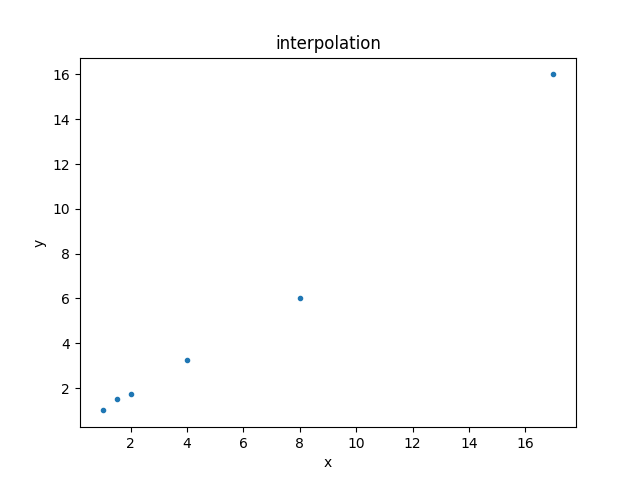## Setting up the model

For this example, a second-order polynomial is going to be fit to the data by using the Polynom1D class:

>>> from sherpa.models.basic import Polynom1D
>>> mdl = Polynom1D()
>>> print(mdl)
polynom1d
Param        Type          Value          Min          Max      Units
-----        ----          -----          ---          ---      -----
polynom1d.c0 thawed            1 -3.40282e+38  3.40282e+38
polynom1d.c1 frozen            0 -3.40282e+38  3.40282e+38
polynom1d.c2 frozen            0 -3.40282e+38  3.40282e+38
polynom1d.c3 frozen            0 -3.40282e+38  3.40282e+38
polynom1d.c4 frozen            0 -3.40282e+38  3.40282e+38
polynom1d.c5 frozen            0 -3.40282e+38  3.40282e+38
polynom1d.c6 frozen            0 -3.40282e+38  3.40282e+38
polynom1d.c7 frozen            0 -3.40282e+38  3.40282e+38
polynom1d.c8 frozen            0 -3.40282e+38  3.40282e+38
polynom1d.offset frozen            0 -3.40282e+38  3.40282e+38


The help for Polynom1D shows that the model is defined as:

$f(x) = \sum_{i=0}^8 c_i (x - {\rm offset})^i$

so to get a second-order polynomial we have to thaw the c2 parameter (the linear term c1 is kept at 0 to show that the choice of parameter to fit is up to the user):

>>> mdl.c2.thaw()


This model can be compared to the data using the ModelPlot class (note that, unlike the data plot, the prepare() method takes both the data - needed to know what $$x_i$$ to use - and the model):

>>> from sherpa.plot import ModelPlot
>>> mplot = ModelPlot()
>>> mplot.prepare(d, mdl)
>>> dplot.plot()
>>> mplot.overplot()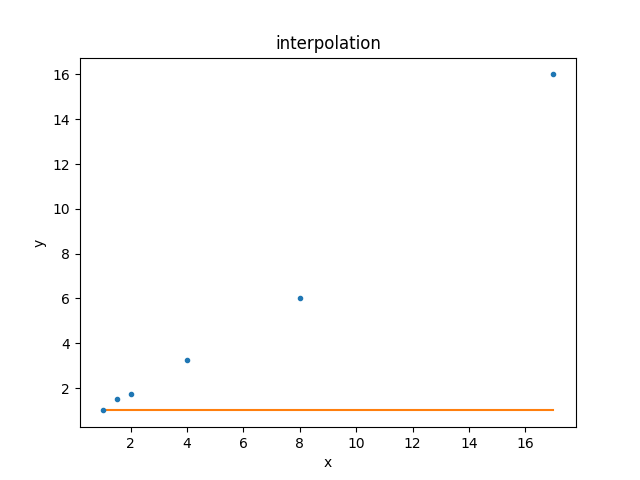Since the default parameter values are still being used, the result is not a good description of the data. Let’s fix this!

## Fitting the model to the data

Since we have no error bars, we are going to use least-squares minimisation - that is, minimise the square of the distance between the model and the data using the LeastSq statisic and the NelderMead optimiser (for this case the LevMar optimiser is likely to produce as good a result but faster, but I have chosen to select the more robust method):

>>> from sherpa.stats import LeastSq
>>> from sherpa.fit import Fit
>>> f = Fit(d, mdl, stat=LeastSq(), method=NelderMead())
>>> print(f)
data      = interpolation
model     = polynom1d
stat      = LeastSq
estmethod = Covariance


In this case there is no need to change any of the options for the optimiser (the least-squares statistic has no options), so the objects are passed straight to the Fit object.

The fit() method is used to fit the data; as it returns useful information (in a FitResults object) we capture this in the res variable, and then check that the fit was succesfull (i.e. it converged):

>>> res = f.fit()
>>> res.succeeded
True


For this example the time to perform the fit is very short, but for complex data sets and models the call can take a long time!

A quick summary of the fit results is available via the format() method, while printing the variable retutrns more details:

>>> print(res.format())
Statistic             = leastsq
Initial fit statistic = 255.875
Final fit statistic   = 2.4374 at function evaluation 264
Data points           = 6
Degrees of freedom    = 4
Change in statistic   = 253.438
polynom1d.c0   1.77498
polynom1d.c2   0.0500999
>>> print(res)
datasets       = None
itermethodname = none
statname       = leastsq
succeeded      = True
parnames       = ('polynom1d.c0', 'polynom1d.c2')
parvals        = (1.7749826216226083, 0.050099944904353017)
statval        = 2.4374045728256455
istatval       = 255.875
dstatval       = 253.43759542717436
numpoints      = 6
dof            = 4
qval           = None
rstat          = None
message        = Optimization terminated successfully
nfev           = 264


The best-fit parameter values can also be retrieved from the model itself:

>>> print(mdl)
polynom1d
Param        Type          Value          Min          Max      Units
-----        ----          -----          ---          ---      -----
polynom1d.c0 thawed      1.77498 -3.40282e+38  3.40282e+38
polynom1d.c1 frozen            0 -3.40282e+38  3.40282e+38
polynom1d.c2 thawed    0.0500999 -3.40282e+38  3.40282e+38
polynom1d.c3 frozen            0 -3.40282e+38  3.40282e+38
polynom1d.c4 frozen            0 -3.40282e+38  3.40282e+38
polynom1d.c5 frozen            0 -3.40282e+38  3.40282e+38
polynom1d.c6 frozen            0 -3.40282e+38  3.40282e+38
polynom1d.c7 frozen            0 -3.40282e+38  3.40282e+38
polynom1d.c8 frozen            0 -3.40282e+38  3.40282e+38
polynom1d.offset frozen            0 -3.40282e+38  3.40282e+38


as can the current fit statistic (as this is for fitting a second-order polynomial I’ve chosen to label the variable with a suffix of 2, which will make more sense below):

>>> stat2 = f.calc_stat()
>>> print("Statistic = {:.4f}".format(stat2))
Statistic = 2.4374


Note

In an actual analysis session the fit would probably be repeated, perhaps with a different optimiser, and starting from a different set of parameter values, to give more confidence that the fit has not been caught in a local minimum. This example is simple enough that this is not needed here.

To compare the new model to the data I am going to use a FitPlot - which combines a DataPlot and ModelPlot - and a ResidPlot - to look at the residuals, defined as $${\rm data}_i - {\rm model}_i$$, using the SplitPlot class to orchestrate the display (note that mplot needs to be re-created since the model has changed since the last time its prepare method was called):

>>> from sherpa.plot import FitPlot, ResidPlot, SplitPlot
>>> fplot = FitPlot()
>>> mplot.prepare(d, mdl)
>>> fplot.prepare(dplot, mplot)
>>> splot = SplitPlot()
>>> rplot = ResidPlot()
>>> rplot.prepare(d, mdl, stat=LeastSq())
WARNING: The displayed errorbars have been supplied with the data or calculated using chi2xspecvar; the errors are not used in fits with leastsq
>>> rplot.plot_prefs['yerrorbars'] = False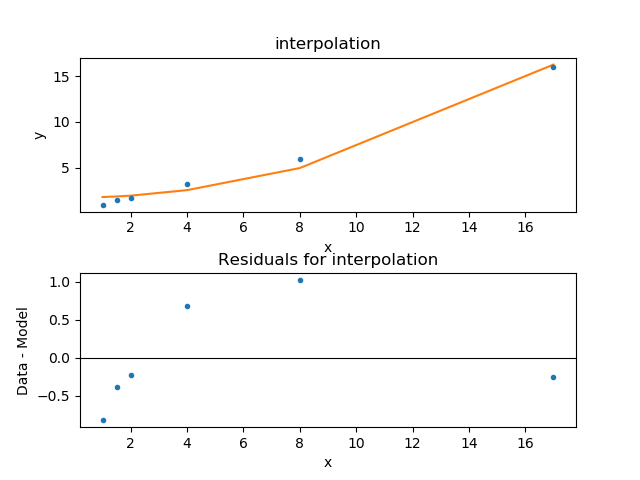The default behavior for the residual plot is to include error bars, here calculated using the Chi2XspecVar class, but they have been turned off - by setting the yerrorbars option to False - since they are not meaningful here.

## Interpolating values

The model can be evaluated directly by supplying it with the independent-axis values; for instance for $$x$$ equal to 2, 5, and 10:

>>> print(mdl([2, 5, 10]))
[1.9753824  3.02748124 6.78497711]


It can also be used to extrapolate the model outside the range of the data (as long as the model is defined for these values):

>>> print(mdl([-100]))
[502.77443167]
>>> print(mdl([234.56]))
[2758.19347071]


## Changing the fit

Let’s see how the fit looks if we use a linear model instead. This means thawing out the c1 parameter and clearing c2:

>>> mdl.c1.thaw()
>>> mdl.c2 = 0
>>> mdl.c2.freeze()
>>> f.fit()
<Fit results instance>


As this is a simple case, I am ignoring the return value from the fit() method, but in an actual analysis session it should be checked to ensure the fit converged.

The new model parameters are:

>>> print(mdl)
polynom1d
Param        Type          Value          Min          Max      Units
-----        ----          -----          ---          ---      -----
polynom1d.c0 thawed    -0.248624 -3.40282e+38  3.40282e+38
polynom1d.c1 thawed     0.925127 -3.40282e+38  3.40282e+38
polynom1d.c2 frozen            0 -3.40282e+38  3.40282e+38
polynom1d.c3 frozen            0 -3.40282e+38  3.40282e+38
polynom1d.c4 frozen            0 -3.40282e+38  3.40282e+38
polynom1d.c5 frozen            0 -3.40282e+38  3.40282e+38
polynom1d.c6 frozen            0 -3.40282e+38  3.40282e+38
polynom1d.c7 frozen            0 -3.40282e+38  3.40282e+38
polynom1d.c8 frozen            0 -3.40282e+38  3.40282e+38
polynom1d.offset frozen            0 -3.40282e+38  3.40282e+38


and the best-fit statistic value can be compared to the earlier version:

>>> stat1 = f.calc_stat()
>>> print("Statistic: order 1 = {:.3f} order 2 = {:.3f}".format(stat1, stat2))
Statistic: order 1 = 1.898 order 2 = 2.437


Note

Sherpa provides several routines for comparing statistic values, such as sherpa.utils.calc_ftest() and sherpa.utils.calc_mlr(), to see if one can be preferred over the other, but these are not relevant here, as the statistic being used is just the least-squared difference.

The two models can be visually compared by taking advantage of the previous plot objects retaining the values from the previous fit:

>>> mplot2 = ModelPlot()
>>> mplot2.prepare(d, mdl)
>>> mplot.plot()
>>> mplot2.overplot()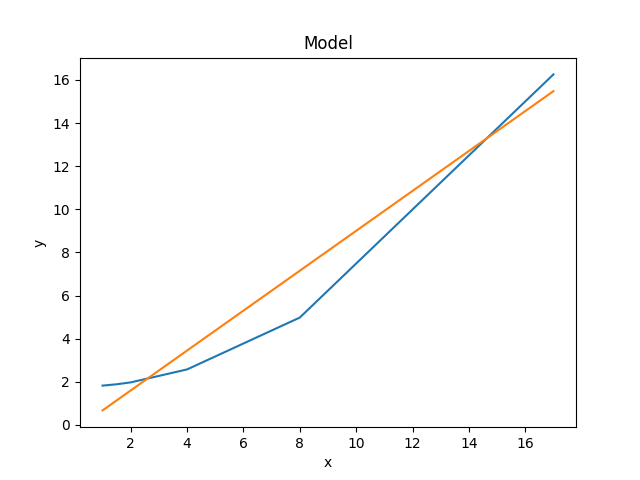An alternative would be to create the plots directly (the order=2 parameter values are restored from the res object created from the first fit to the data), in which case we are not limited to calculating the model on the independent axis of the input data (the order is chosen to match the colors of the previous plot):

>>> xgrid = np.linspace(0, 20, 21)
>>> y1 = mdl(xgrid)
>>> mdl.c0 = res.parvals
>>> mdl.c1 = 0
>>> mdl.c2 = res.parvals
>>> y2 = mdl(xgrid)
>>> plt.clf()
>>> plt.plot(xgrid, y2, label='order=2');
>>> plt.plot(xgrid, y1, label='order=1');
>>> plt.legend();
>>> plt.title("Manual evaluation of the models");